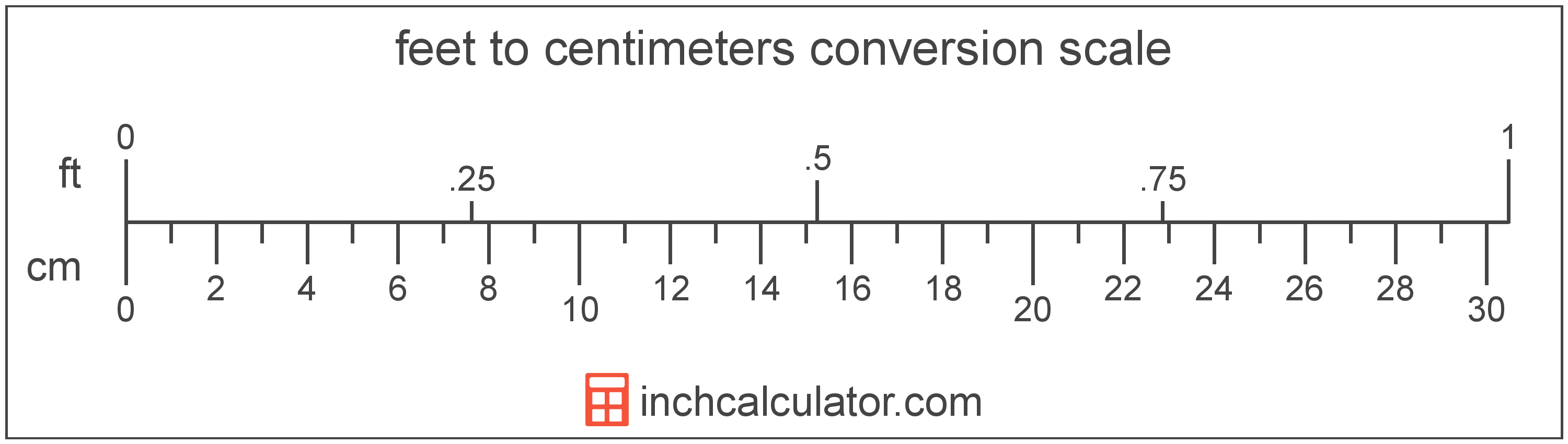# Feet to Centimeters Conversion

Enter the length in feet below to get the value converted to centimeters.

ft
in
Results in Centimeters:1 ft = 30.48 cm
Do you want to convert centimeters to feet?

## How to Convert Feet to CentimetersTo convert a foot measurement to a centimeter measurement, multiply the length by the conversion ratio.

Since one foot is equal to 30.48 centimeters, you can use this simple formula to convert:

centimeters = feet × 30.48

The length in centimeters is equal to the feet multiplied by 30.48.

For example, here's how to convert 5 feet to centimeters using the formula above.
5' = (5 × 30.48) = 152.4 cm

Our inch fraction calculator can add feet and centimeters together, and it also automatically converts the results to US customary, imperial, and SI metric values.

## Feet

The foot is a unit of linear length measure equal to 12 inches or 1/3 of a yard. Because the international yard is legally defined to be equal to exactly 0.9144 meters, one foot is equal to 0.3048 meters.

The foot is a US customary and imperial unit of length. Feet can be abbreviated as ft; for example, 1 foot can be written as 1 ft.

Feet can also be denoted using the symbol, otherwise known as a prime, though a single-quote (') is often used instead of the prime symbol for convenience. Using the prime symbol, 1 ft can be written as 1′.

The foot is most commonly measured using either a standard 12" ruler or a tape measure, though there are many other measuring devices available. Feet are sometimes referred to as linear feet, which are simply the measurement of length in feet.

You might be interested in our feet and inches calculator, which can add feet with inches, centimeters, or meters.

## Centimeters

One centimeter is equal to one-hundredth (1/100) of a meter, which is defined as the distance light travels in a vacuum in a 1/299,792,458 second time interval.

The centimeter, or centimetre, is a multiple of the meter, which is the SI base unit for length. In the metric system, "centi" is the prefix for 10-2. Centimeters can be abbreviated as cm; for example, 1 centimeter can be written as 1 cm.

Metric rulers typically have 30 cm, which are represented by 30 large tick marks. To get a rough idea of the actual length of a centimeter, a standard pencil is just about 1 cm thick.

We recommend using a ruler or tape measure for measuring length, which can be found at a local retailer or home center. Rulers are available in imperial, metric, or combination with both values, so make sure you get the correct type for your needs.

Need a ruler? Try our free downloadable and printable rulers, which include both imperial and metric measurements.

## Foot to Centimeter Conversion Table

Foot measurements converted to centimeters
Feet Centimeters
1' 30.48 cm
2' 60.96 cm
3' 91.44 cm
4' 121.92 cm
5' 152.4 cm
6' 182.88 cm
7' 213.36 cm
8' 243.84 cm
9' 274.32 cm
10' 304.8 cm
11' 335.28 cm
12' 365.76 cm
13' 396.24 cm
14' 426.72 cm
15' 457.2 cm
16' 487.68 cm
17' 518.16 cm
18' 548.64 cm
19' 579.12 cm
20' 609.6 cm
21' 640.08 cm
22' 670.56 cm
23' 701.04 cm
24' 731.52 cm
25' 762 cm
26' 792.48 cm
27' 822.96 cm
28' 853.44 cm
29' 883.92 cm
30' 914.4 cm
31' 944.88 cm
32' 975.36 cm
33' 1,006 cm
34' 1,036 cm
35' 1,067 cm
36' 1,097 cm
37' 1,128 cm
38' 1,158 cm
39' 1,189 cm
40' 1,219 cm

## References

1. National Institute of Standards and Technology, U.S. Survey Foot: Revised Unit Conversion Factors, https://www.nist.gov/pml/us-surveyfoot/revised-unit-conversion-factors